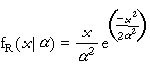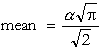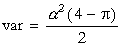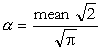Rayleigh Distribution

#Rayleigh Distribution

The Rayleigh Distribution, also known as the Non-Central Chi-Squared Distribution, is most commonly used in mathematical physics, especially in communication theory. It is also used to represent the distribution of a sample of variance from a normal population with an unstable expected value.

The Rayleigh Distribution is defined for all positive values of x. It has one parameter, a, which must be positive. The distribution is:where x>0 and a>0.

The equations for the mean and variance are:The equation for the parameter is:The mean must be positive.

Versions: DPL Professional, DPL Enterprise, DPL Portfolio